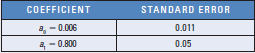# Testing for a Forecast Bias You must determine whether there is a forecast bias in the forward rate. You apply regression analysis to test the relationship between the actual spot rate and the forward rate forecast ( F ): The regression results are as follows: Based on these results, is there a bias in the forecast? Verify your conclusion. If there is a bias, explain whether it is an overestimate or an underestimate.FindFind

### International Financial Management

14th Edition
Madura
Publisher: Cengage
ISBN: 9780357130698FindFind

### International Financial Management

14th Edition
Madura
Publisher: Cengage
ISBN: 9780357130698

#### Solutions

Chapter 9, Problem 20QA
Textbook Problem

## Testing for a Forecast Bias You must determine whether there is a forecast bias in the forward rate. You apply regression analysis to test the relationship between the actual spot rate and the forward rate forecast (F):The regression results are as follows:Based on these results, is there a bias in the forecast? Verify your conclusion. If there is a bias, explain whether it is an overestimate or an underestimate.

This textbook solution is under construction.

## Expert Solution

### Want to see the full answer?

Check out a sample textbook solution.

### Want to see this answer and more?

Experts are waiting 24/7 to provide step-by-step solutions in as fast as 30 minutes!*

*Response times vary by subject and question complexity. Median response time is 34 minutes and may be longer for new subjects.© 2021 bartleby. All Rights Reserved.